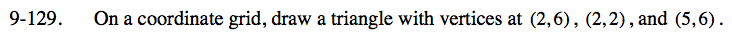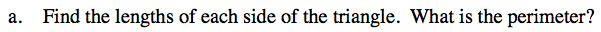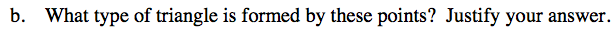Home > ACC7 > Chapter cc39 > Lesson cc39.2.5 > Problem9-129

9-129.
1. On a coordinate grid, draw a triangle with vertices at (2, 6), (2, 2), and (5, 6). 9-129 HW eTool (Desmos). Homework Help ✎

1. Find the lengths of each side of the triangle. What is the perimeter?

2. What type of triangle is formed by these points? Justify your answer.To find the hypotenuse, we can use the Pythagorean theorem: a2 + b2 = c2
If the legs are 3 and 4, how long is the hypotenuse?
After finding the hypotenuse, find the perimeter.The triangle has a right angle so it is a right triangle.

Use the eTool below to help you with this problem.
Click the link at right for the full version of the eTool: CC3 9-129 HW eTool